Browse by Topic

# Class Notes, Quizzes and Weather Alerts with Mathematica and the Wolfram Language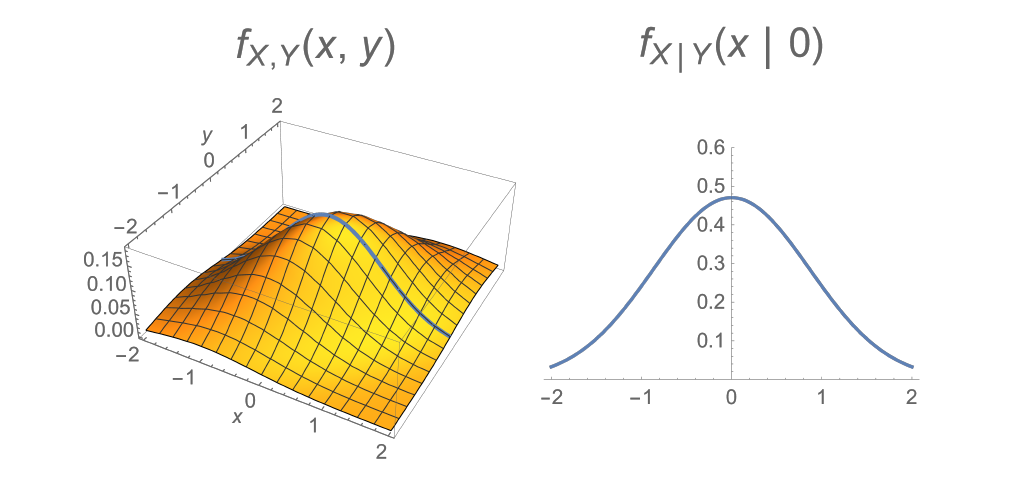Using Wolfram technologies has always been a part of my working processfrom asking Wolfram|Alpha questions in college to using the Wolfram Cloud to set up reminders and forms in my everyday work. Nowadays, I think about the ways that our users can employ our technologies. I like to build on things that I perhaps should have used to improve my efficiency during my time as a student or faculty member, or even for tasks outside of work in my day-to-day life.

## Class Work and Taking Notes

One thing I wanted to get better at as a student was “live TeXing” notes during a class or seminar. (That is, writing my notes in LaTeX while a presentation was actively happening.) Many times I missed getting the right information or lost the thread of the discussion while trying to find the right formatting in my LaTeX commands.

Recently I’ve been taking part in a series of lectures from the London Mathematical Society’s online lecture series covering model theory, which is a branch of logic that studies the first-order sentences in different structures, as well as a probability course from the MicroMasters Program in Statistics and Data Science from the Massachusetts Institute of Technology (MIT). I use Mathematica notebooks to take notes and make concepts clear for myself and others in those classes.

The Wolfram Language’s typesetting abilities include a wide array of special characters and different ways of being able to input them. This includes using either the named character input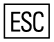characteror the brackets notation \[character], which make it easy to type fast and effectively while taking notes during a course.

Here are a couple of snippets from my notes made during a model theory lecture: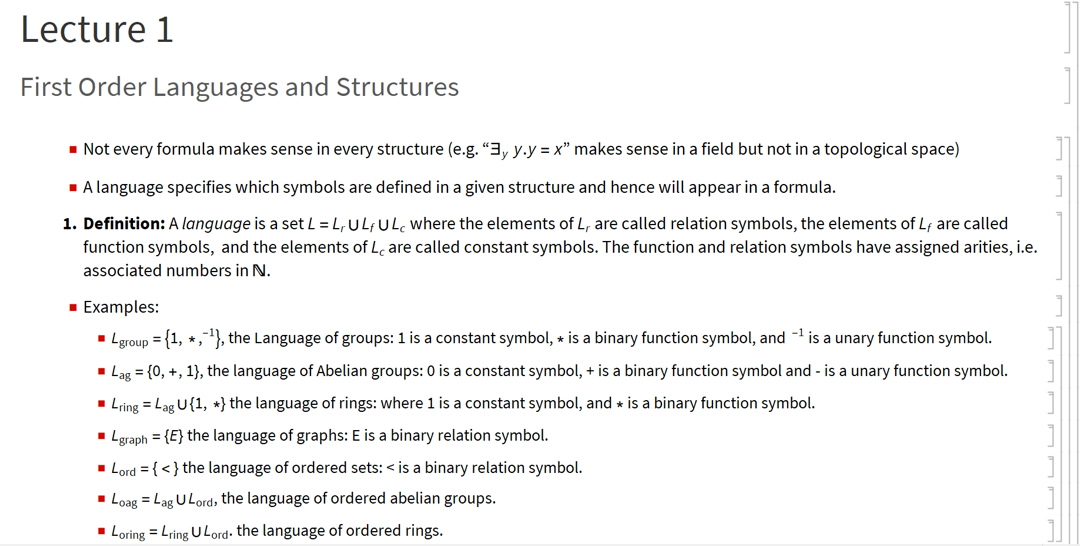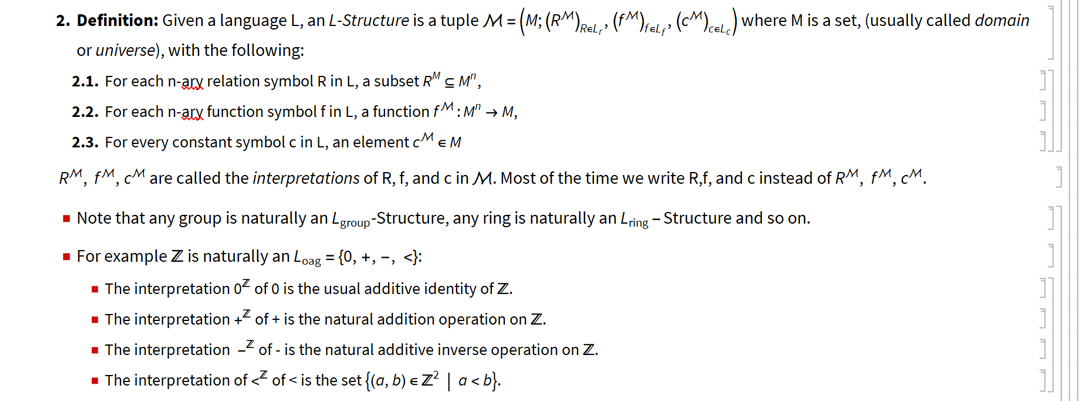I built the following Manipulate to graphically show the relationship between conditional probability density functions arising from a joint probability distribution. As you move along the y axis, you obtain a different probability distribution for the conditional random variable X conditioned on Y = y: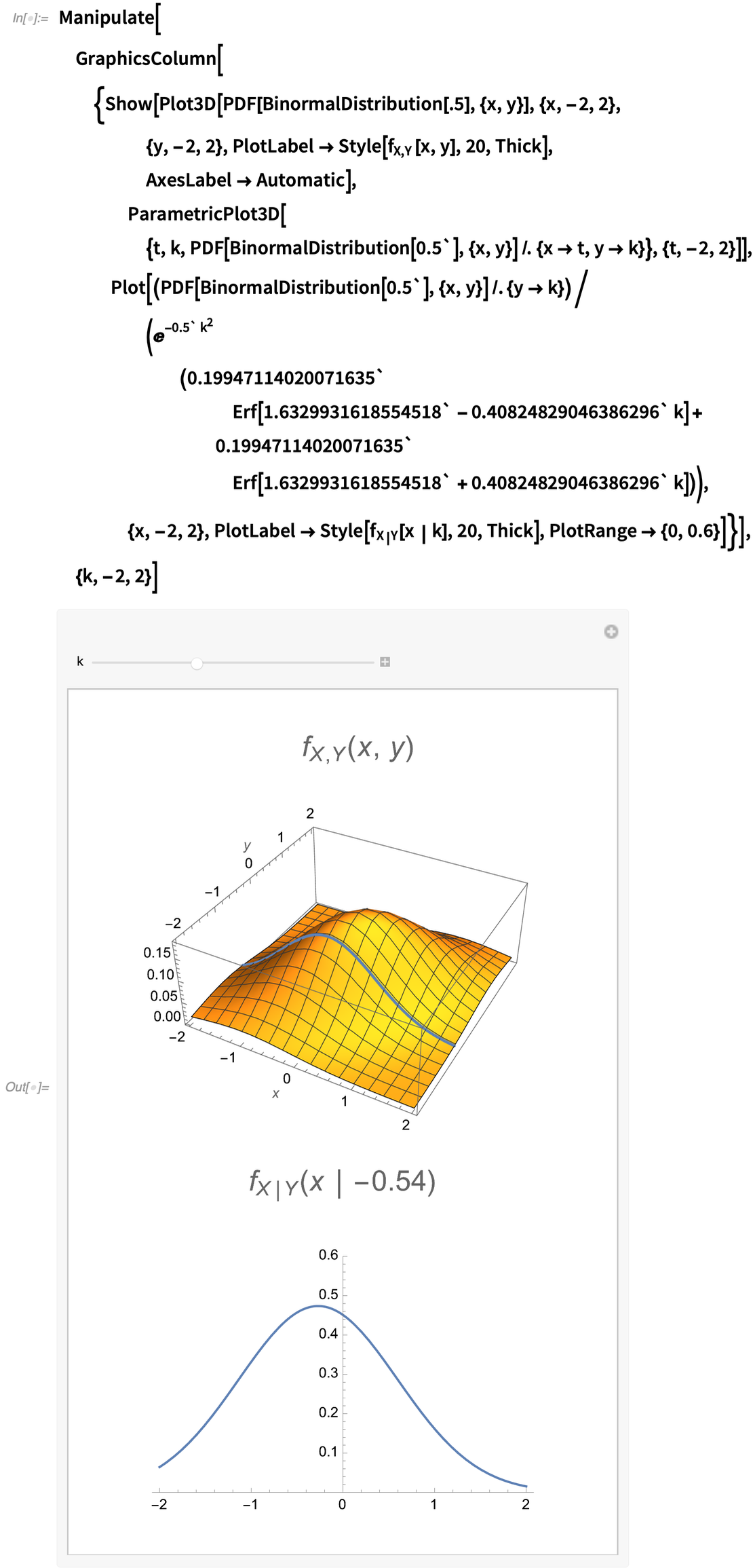✕ Manipulate[ GraphicsColumn[{Show[ Plot3D[ PDF[BinormalDistribution[.5], {x, y}], {x, -2, 2}, {y, -2, 2}, PlotLabel -> Style[Subscript[f, X, Y][x, y], 20, Thick], AxesLabel -> Automatic], ParametricPlot3D[{t, k, PDF[BinormalDistribution[0.5], {x, y}] /. {x -> t, y -> k}}, {t, -2, 2}]], Plot[(PDF[ BinormalDistribution[0.5], {x, y}] /. {y -> k})/(E^(-0.5 k^2) (0.19947114020071635 Erf[ 1.6329931618554518 - 0.40824829046386296 k] + 0.19947114020071635 Erf[ 1.6329931618554518 + 0.40824829046386296 k])), {x, -2, 2}, PlotLabel -> Style[Subscript[f, X | Y][x | k], 20, Thick], PlotRange -> {0, 0.6}]}], {k, -2, 2}]

In general, using notebooks for note taking and visual aids has helped me stay focused and clarify ideas present in different settings.

As a teacher, I found myself having a rough time with grading student work and tests. It is very important to assess whether students have learned the class material (both for the sake of the students and the instructor), but it’s also a very time-consuming process. Wolfram has production-level solutions for the creation of assessments and grading, and our Technical Consulting team can customize applications such as Wolfram Problem Generator for specific classes.

To give an example of a small-scale solution, I transformed some quiz/exam questions from my classes into a form and deployed it to the Wolfram Cloud. Sharing the form was very easy and provided the assessment grade to students as soon as they submitted their completed forms. This is a small but handy example for using the Wolfram Language for online and remote learning—a feature that proved itself to be incredibly important when COVID-19 precautions required students and teachers to transition to online and hybrid classrooms.

For projects of this kind, I use FormFunction or FormPage and CloudDeploy. The general setup is CloudDeploy[FormFunction[formQuestions,function],"name of cloud object"], where formQuestions is the part that controls the questions and the different fill options, and function is the code that tells the form what to do with the information that someone has submitted.

The following code sample shows how you can deploy a typical quiz with a true-or-false section and two computations. After a student completes the assessment and submits the form, it will show the student’s score and store it with the person’s name in a Databin in the Wolfram Data Drop: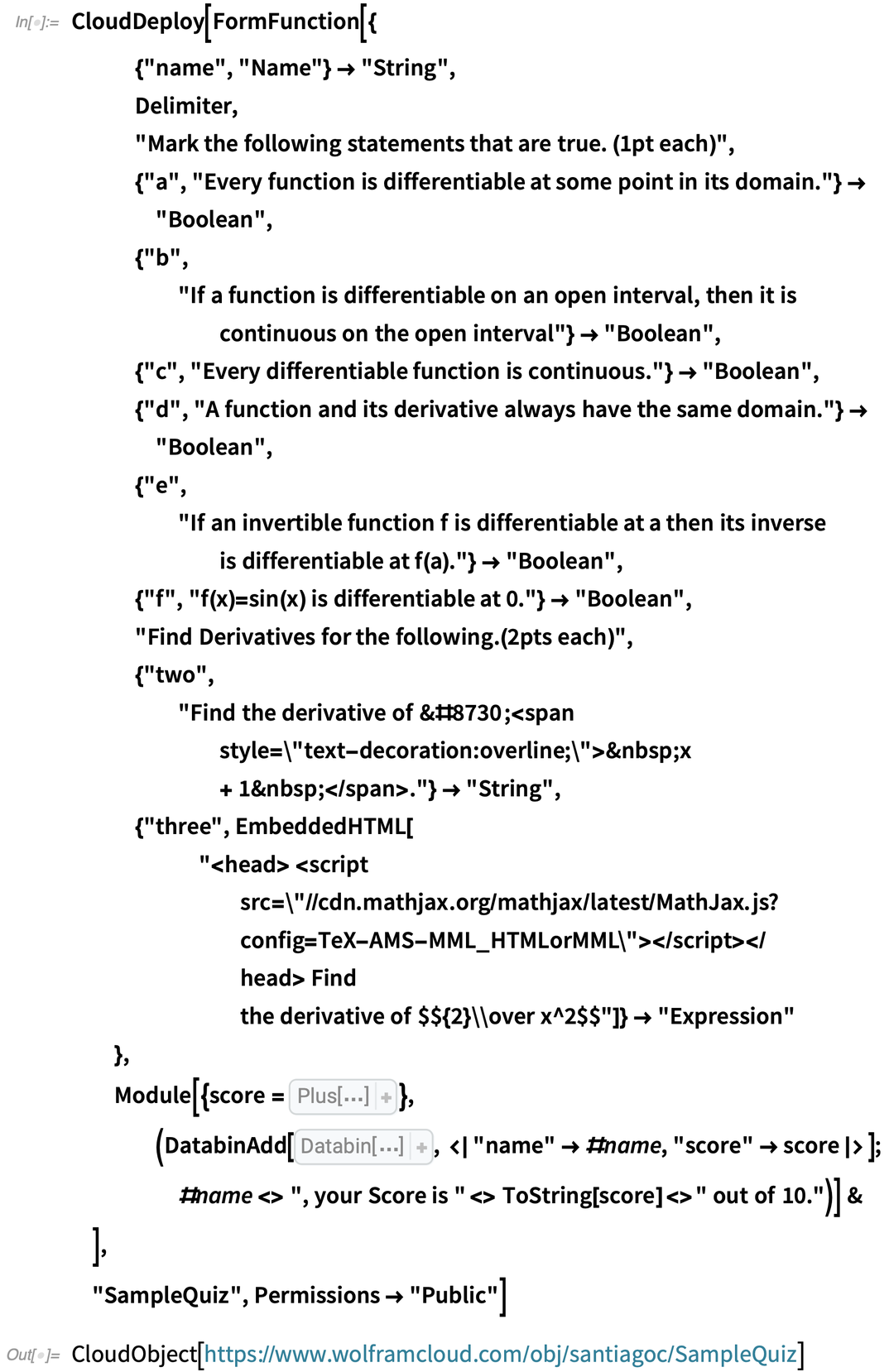✕ CloudDeploy[FormFunction[{ {"name", "Name"} -> "String", Delimiter, "Mark the following statements that are true. (1pt each)", {"a", "Every function is differentiable at some point in its domain."} \ -> "Boolean", {"b", "If a function is differentiable on an open interval, then it is \ continuous on the open interval"} -> "Boolean", {"c", "Every differentiable function is continuous."} -> "Boolean", {"d", "A function and its derivative always have the same domain."} -> "Boolean", {"e", "If an invertible function f is differentiable at a then its \ inverse is differentiable at f(a)."} -> "Boolean", {"f", "f(x)=sin(x) is differentiable at 0."} -> "Boolean", "Find Derivatives for the following.(2pts each)", {"two", "Find the derivative of √ x + 1 ."} -> "String", {"three", EmbeddedHTML[ " Find the derivative of $${2}\\over \ x^2$$"]} -> "Expression" }, Module[{score = Boole[ Not[ Slot["a"]]] + Boole[ Slot["b"]] + Boole[ Slot["c"]] + Boole[ Not[ Slot["d"]]] + Boole[ Slot["e"]] + Boole[ Slot["f"]] + 2 Boole[ FullSimplify[ToExpression[ Slot["two"]] === 1/(2 Sqrt[1 + x])]] + 2 Boole[ FullSimplify[Slot["three"] === -(4/x^3)]]}, (DatabinAdd[ CloudGet["http://wolfr.am/VWFFyE3A"], <|"name" -> #name, "score" -> score|>]; #name <> ", your Score is " <> ToString[score] <> " out of 10.")] & ], "SampleQuiz", Permissions -> "Public"]

(For full disclosure, the code is a bit fragile. It may not run, depending on the input provided as a response to the open questions. You can strengthen the code by using a few error-control additions, but this example is meant to be only a quick project, so I wanted to keep the code relatively simple.)

The most time-consuming aspect of this project was figuring out how to display the math nicely in the cloud-deployed form (specifically in the questions about the derivative of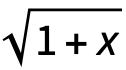and the derivative of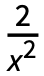). I’m used to the typesetting found in desktop notebooks, but eventually I found two ways to work with the browser interface for this particular issue.

The first solution is done by constructing the square root using its HTML entity (&radic;) and overlining the remainder of the expression. This solution seems hacky, however, and overly specific to this test type.

The second solution I came up with uses the EmbeddedHTML function, which allowed me to add MathJax to eventually use LaTeX, which in my opinion is more straightforward. I left both methods in the previous code example for comparison.

Since moving to Urbana, Illinois, in the Midwest from Colombia, the hardest thing for me has been dealing with snow. Based on an idea from Stephen Wolfram’s Introduction to the Wolfram Language video, I built a scheduler to ask Wolfram|Alpha if it is going to snow in my area the following day. If so, it sends me an email notification. That way I can prepare both physically (for example by setting alarms earlier to have time to defrost the car if necessary) and mentally.

The code is even shorter here. I have a function for sending a snow alert to an email address together with the link to the Wolfram|Alpha query so I can see what prompted it. I’ve slightly modified it from the one I actually used because in the original, I used my personal email: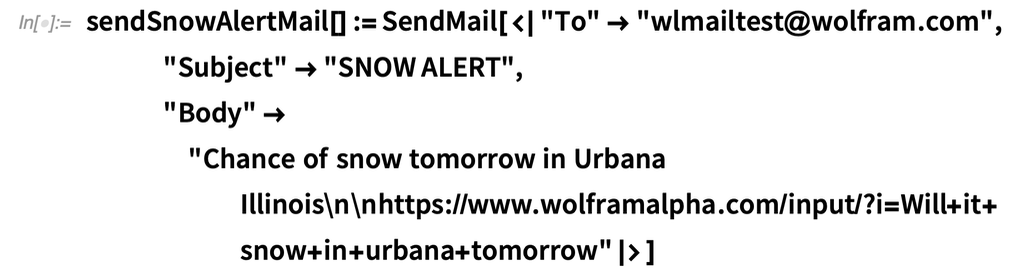✕ sendSnowAlertMail[] := SendMail[<|"To" -> "wlmailtest@wolfram.com", "Subject" -> "SNOW ALERT", "Body" -> "Chance of snow tomorrow in Urbana Illinois\n\n\ https://www.wolframalpha.com/input/?i=Will+it+snow+in+urbana+\ tomorrow"|>]

Then I have a function that checks for snow by pinging Wolfram|Alpha and calling the previous function if it shows that it will snow: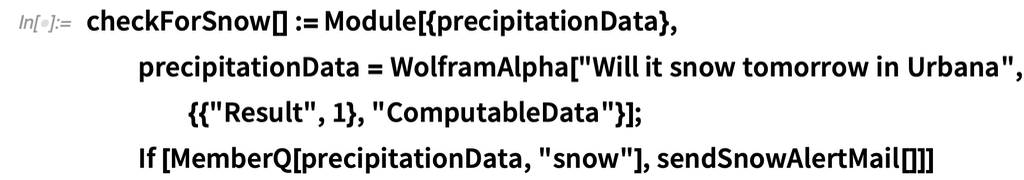✕ checkForSnow[] := Module[{precipitationData}, precipitationData = WolframAlpha[ "Will it snow tomorrow in Urbana", {{"Result", 1}, "ComputableData"}]; If [MemberQ[precipitationData, "snow"], sendSnowAlertMail[]]]

I then save the checkForSnow function in a cloud object: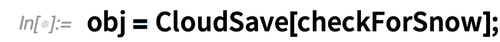✕ obj = CloudSave[checkForSnow];

Finally, I deploy a ScheduledTask to run the checkForSnow function every day at 5pm: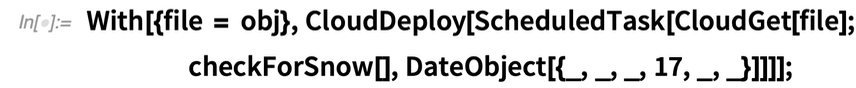✕ With[{file = obj}, CloudDeploy[ ScheduledTask[CloudGet[file]; checkForSnow[], DateObject[{_, _, _, 17, _, _}]]]];`

It has proven quite useful. Of course, I could always look daily at tomorrow’s weather forecast, but for the most part, only snow really changes my routine, and this setup saves me time I can spend on other activities.

We have been fortunate that it has not snowed in Urbana since April, so this is what the most recent email I got looked like: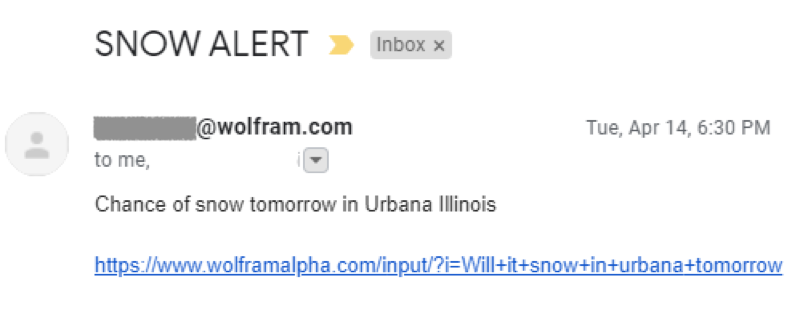Of course we can add bells and whistles to improve the email’s style with things such as EmbeddedHTML, but for my purposes, the simple-looking email is enough.

## More Projects in the Works

I always have several Mathematica notebooks open while working on my computer, whether they are for answering questions for customers, running code for a colleague, preparing presentations, analyzing data, running personal projects or even writing this blog! I enjoy how I can use the same platform for a variety of different tasks, and I like how easy it is to take a random idea I think of and turn it into something more.

I would be happy to hear about any other projects people are involved in, so please do not hesitate to reach out and email me directly with your projects/ideas or questions about how to leverage the Wolfram Language in them.

 Get full access to the latest Wolfram Language functionality with a Mathematica 12.3 or Wolfram|One trial.